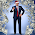Tuesday, March 31, 2015

Preview (and correction notice): Derivation MpRp=4LM #5

An update to the derivation is needed to include the information theory part as mentioned in Derivation MpRp=4LM #3. After reviewing Nassim's paper again,

Haramein, N. (2012). Quantum Gravity and the Holographic Mass, Physical Review & Research International, ISSN: 2231-1815, Page 270-292

a more direct approach is required, which is basically detailing the steps in his paper and examining the justification of each step.

The mass of the black hole is geometrically and informational theory related to the Planck mass

mh = (R/η)ml
mh = Mass of Black Hole
R = V/Vl
η = As/Al
ml = Planck Mass

V = Volume of the interior of the black hole
As = Area of black hole surface
Vl = Planck spherical unit volume
Al =  Area, equatorial cross-sectional area

Let's see were we go with this next. Things may not have been so simple as I first implied, however, we are going to go with it, to proceed, to see the simplicity in how the equations match observation and then do our best to derive and understand each step.

It's looks like it's time for a good mathematical symbol add on as well...

It's time to get back on the right track!!!  (4:19) <--Boom, pow! (a video link)

More later,
The Surfer, OM-IV

PS: The Surfer's flounder with Derivation of MpRp=4LM #4 will be instrumental in gaining insight into the final resolution to this issue of how to calculate the mass of a black hole and what it's mass.radius relationship is.  It still has something to do with this density of the vacuum of space equation T and the radius of the spherical volume of the inside of the black hole.  No Fear of mistakes, because from mistakes comes great knowledge and experience, great teachings, if one lives through it... or possibly even if one does not...

"It's the η that'll gate cha!" - Master Moe

1.A little look ahead I'd that the T=M/V is only part of the solution. What we will find is the answer included fundamental Planck Units, and the ratio of two "large number" ratios. Now we know the significant of large numbers ratios found in science. Links later to this large number ratio stuff...

1.2.3.